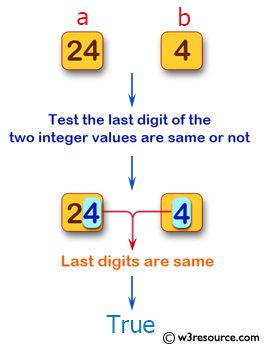﻿ Swift Basic Programming Exercise: Test whether the last digit of the two given non-negative integer values are same or not - w3resource# Swift Basic Programming Exercise: Test whether the last digit of the two given non-negative integer values are same or not

## Swift Basic Programming: Exercise-18 with Solution

Write a Swift program to test whether the last digit of the two given non-negative integer values are same or not.

Pictorial Presentation:Sample Solution:

Swift Code:

``````func same_last_Digit(_ a: Int, _ b: Int) -> Bool {
guard a < 0, b < 0
else
{
if a % 10 == b % 10
{
return true
}
else
{
return false
}
}
return false
}
print(same_last_Digit(3, 13))
print(same_last_Digit(24, 4))
print(same_last_Digit(12, 24))
```
```

Sample Output:

```true
true
false
```

Swift Programming Code Editor:

Improve this sample solution and post your code through Disqus

What is the difficulty level of this exercise?

﻿